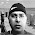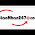## Sunday, September 9, 2012

### Generating all permutations, combinations, and power set of a string (or set of numbers)

Combinatorics is a branch of mathematics that deal with counting of discrete structures. Two concepts that often come up in the study of combinatorics are permutaions and combinations of a set of discrete elements.

The number of possible permutations (and combinations) that can be generated from a set of discrete elements can be huge. We will leave the mathematical analysis here and instead focus on how to generate all permutations and combinations of a set of numbers/characters by using a computer program. We will be using the Java programming language to write the code for this.

A related concept is the concept of Power Set. A Power Set of a set of discrete elements is the set of all subsets of elements from the original elements. Essentially this is a set of all combinations of all lengths (0 to size of the set) of the set.

Generating all permutations of a string (of characters):

The key to understanding how we can generate all permutations of a given string is to imagine the string (which is essentially a set of characters) as a complete graph where the nodes are the characters of the string. This basically reduces the permutations generating problem into a graph traversal problem: given a complete graph, visit all nodes of the graph without visiting any node twice. How many different ways are there to traverse such a graph?

It turns out, each different way of traversing this graph is one permutation of the characters in the given string!

We can use Depth First Search (DFS) traversal technique to traverse this graph of characters. The important thing to keep in mind is that we must not visit a node twice in any "branch" of the depth-first tree that runs down from a node at the top of the tree to the leaf which denotes the last node in the current "branch".

START
/       |         \
A       B        C
/   \      /  \      /   \
B    C   A  C  A   B
|      |    |     |   |      |
C    B  C   A  B    A

In the above figure, a "branch" is the vertical line that connects all 3 characters. A "branch" is one permutation of the given string. In a recursive (DFS-based) solution the trick is to maintain an array that holds one such "branch" at any given time.

Here is the Java code:

void generatePermutations(char[] arr, int size, char[] branch, int level, boolean[] visited)
{
if (level >= size-1)
{
System.out.println(branch);
return;
}

for (int i = 0; i < size; i++)
{
if (!visited[i])
{
branch[++level] = arr[i];
visited[i] = true;
generatePermutations(arr, size, branch, level, visited);
visited[i] = false;
level--;
}
}
}

The above method can be called like this:

String str = "ABCD";
int n = str.length();
char[] arr = str.toCharArray();
boolean[] visited = new boolean[n];
for (int i = 0; i < n; i++)
visited[i] = false;
char[] branch = new char[n];
generatePermutations(arr, n, branch, -1, visited);

The visited array keeps track of which nodes have been visited already.

Generating combinations of k elements:
Generating combinations of k elements from the given set follows similar algorithm used to generate all permutations, but since we don't want to repeat an a character even in a different order we have to force the recursive calls to not to follow the branches that repeat a set of characters.

If the given string is "ABC" and k = 2, our recursive tree will look like this:

START
/        |
A        B
/     \      |
B      C   C

Here we will have to make sure, once we start a "branch" from a node (character), we must not come back to that node (character) again to start another "branch". So, starting off a new recursive call (to traverse a new "branch") must start from the following node (character)!

Here is the Java code for generating k combinations:

void combine(char[] arr, int k, int startId, char[] branch, int numElem)
{
if (numElem == k)
{
System.out.println(Arrays.toString(branch));
return;
}

for (int i = startId; i < arr.length; ++i)
{
branch[numElem++] = arr[i];
combine(arr, k, ++startId, branch, numElem);
--numElem;
}
}

In the above code, that variable startId makes sure we are never starting a new recursive call for a new "branch". It gets incremented for a new traversal.

To call the combine method above, do this:

int k = 2;
char[] input = "ABCD".toCharArray();
char[] branch = new char[k];
combine(input, k, 0, branch, 0);

Generating the power set:

Generating all subsets (the power set) of a given set of characters (or numbers) is very similar to generating combinations. While generating k-element combinations our goal was to print the current "branch" only when it holds all k characters from the given string. Since a power set contains combination of all lengths, we will simply call combine to generate k combinations for all k where 0 <= k < SIZE(string).

void powerSet(char[] arr)
{
for (int i = 0; i < arr.length; ++i)
{
char[] branch = new char[i];
combine(arr, i, 0, branch, 0);
}
}

To call the powerSet method, simply pass in the character array we want to construct the power set of.

I hope you now have a good idea of how to generate all permutations, k-element combinations, and the power set of a given set of elements. I used to find these really hard in the beginning. But once I have started thinking these in terms of graph traversal problems, things became much easier!

Graph algorithms rock :)

1.This is so cool. I never thought of the permutations problem in terms of graph before. Thanks!

2.Yes, I was never able to fully understand the permutation generation solution until I started viewing this as a graph traversal problem.

3.Nice work. I was just thinking how combos looked like a k-graph problem, searched and found this post. I'd love to see some Big-O here.

4.Thanks Brian! Glad that you liked it. Yeah, I should have added some complexity analysis here. Will try to add that when I have some time.

5.Wow.... Its very Good... Think in very way.... Thanks

6.Exceptional code

7.The combination seems not to be working correctly if the alphabets are repeated in the set. For e.g. for a set ABA the combinations are coming:
[A, B]
[A, A]
[B, A]

Ideally this should be:
[A, B]
[A, A]

8.Hi Golam, thanks for this. but how would you combine elements with a length greater than 1 for instance i have a set {AB, AC, AD, BC, BD, CD} and want to generate all possible unique combinations that have a length of 4 i.e ACBD or BCAD etc. I am having trouble visualizing this as a tree

9.10.11.Amazing! Thank you so much!